# Derivative of Antiderivative (Leibniz Rule) JEE Notes | EduRev

## JEE : Derivative of Antiderivative (Leibniz Rule) JEE Notes | EduRev

The document Derivative of Antiderivative (Leibniz Rule) JEE Notes | EduRev is a part of the JEE Course Mathematics (Maths) Class 12.
All you need of JEE at this link: JEE

Derivative of Antiderivative (Leibnitz Rule)
If h(x) & g(x) are differentiable function of x then,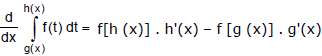Ex.15 Find the derivative of the function g(x) =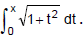Sol. Since f(t) =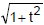is continuous, therefore g'(x) =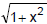Ex.16 If F(t) =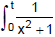dx, find F'(1), F'(2), and F'(x).

Sol. The integrand in this example is the continuous function f defined by f(x) =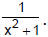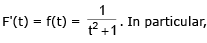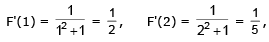Ex.17 Find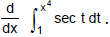Sol. Let u = x4. Then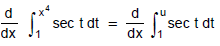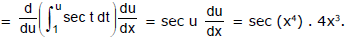Ex.18 FInd the derivative of F(x) =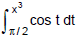Sol.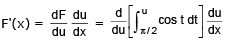= (cos u) (3x2) = (cos x3) (3x2)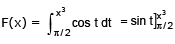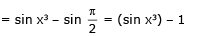F'(x) = (cos x3) (3x2).

Ex.19 Let f(x) =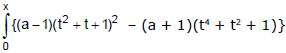. Find the value of 'a' for which f'(x) = 0 has two distinct real roots.

Sol. Differentiating the given equation, we get f'(x) = (a – 1) (x+ x + 1)2 – (a + 1) (x2 + x + 1) (x2 – x + 1).
Now, f'(x) = 0 ⇒ (a – 1) (x2 + x + 1) – (a + 1) (x2 – x + 1) = 0 ⇒ x2 – ax + 1 = 0.
For distinct real roots D > 0 i.e. a2 – 4 > 0 ⇒  a2 > 4  ⇒  a ∈ (-∝, -2) U (2, ∝)

Ex.20 Show that for a differentiable function f(x),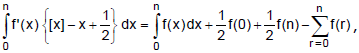(where [ * ] denotes the greaetest integer function and n ε N)

Sol.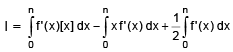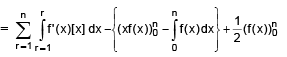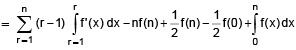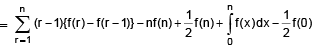= – f(1) – f(2) – ........ – f(n – 1) – f(n)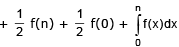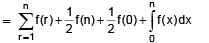Ex.21 Evaluate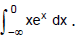Sol.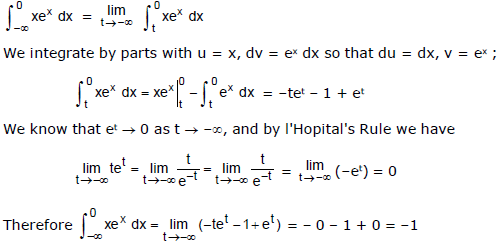Ex.22 Evaluate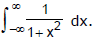Sol.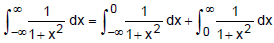We must now evaluate the integrals on the right side separately :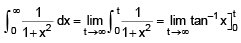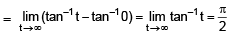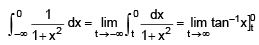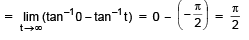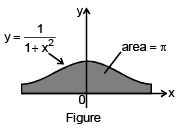Since both of these integrals are convergent, the given integral is convergent and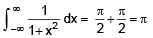Since 1/(1 + x2) > 0, the given improper integral can be interpreated as the area of the infinite region that lies under the curve y = 1/(1 + x2) and above the x-axis (see Figure).

Ex.23 Find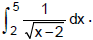Sol.

We note first that the given integral is improper because f(x) = 1/√(x-2) has the vertical asymptote x = 2. Since the infinite discontinuity occurs at the left end point of [2, 5]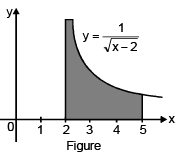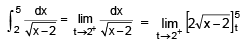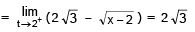Thus, the given improper integrat is convergent and, since the integrand is positive, we can interpret the value of the integral as the area of the shaded region in Figure.

Ex.24 Evaluate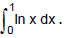Sol. We know that the function f(x) = ln x has a vertical asymptote at 0 since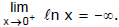Thus, the given integral is improper and we have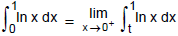Now we integrate by parts with u = ln x, dv = dx, du = dx/x, and v = x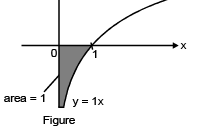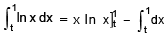=1 ln – t ln t – (1 – t)  = – t ln t – 1 + t

To find the limit of the first term we use I'Hopital's Rule :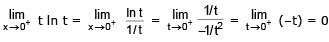Therefore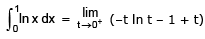= –0 – 1 + 0 = –1

Figure shows the geometric interpretation of this result. The area of the shaded region above y = ln x and below the x-axis is 1.

Ex.25 Evaluate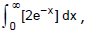(where [ * ] denotes the greatest integer function)

Sol.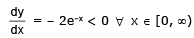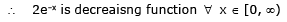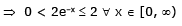for x > ln 2  ⇒  ex > 2 ⇒ e-x < 1/2 ⇒ 2e–x < 1  ∴ 0 ≤ 2e-x < 1 [2e-x] = 0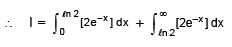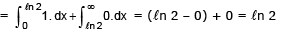Offer running on EduRev: Apply code STAYHOME200 to get INR 200 off on our premium plan EduRev Infinity!

## Mathematics (Maths) Class 12

209 videos|222 docs|124 tests

,

,

,

,

,

,

,

,

,

,

,

,

,

,

,

,

,

,

,

,

,

;